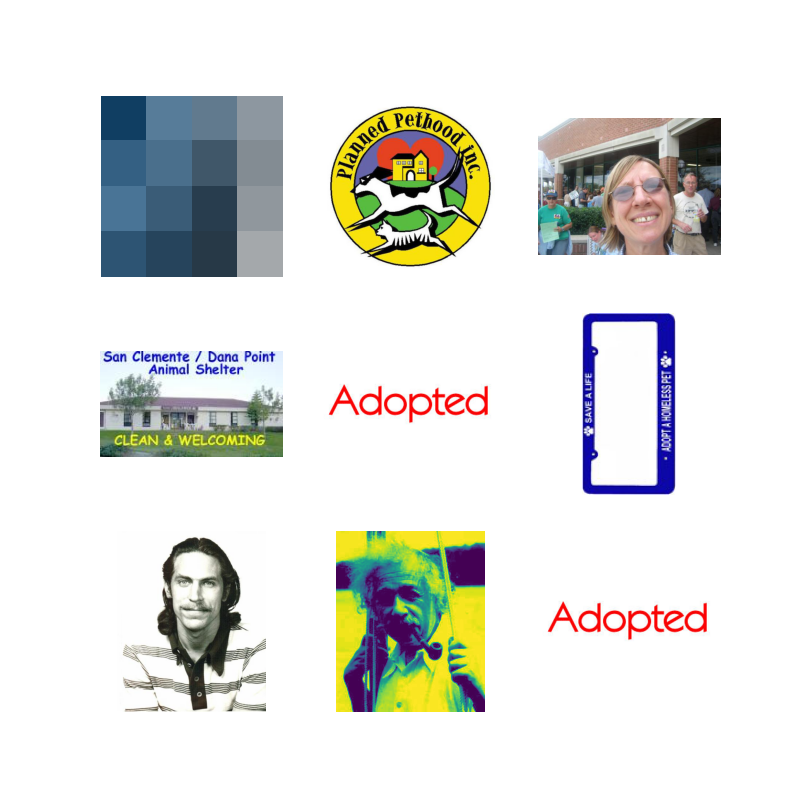# CNN based classification of Cat and Dog WITHOUT transfer learning: 95% validation accuracy¶

Abstract

The purpose of this script is to understand various stages of building a good CNN model. Using transfer learning techniques, one can achieve nearly 100% accuracy for this task. However, in this script I will try to build a model from scratch that leads a reasonable accuracy. The final result shows close to 95% on validation dataset.

Key highlights:

• Create a model from scratch without transfer learning.
• Gain insights on how the depth and width of the model affects the loss curve
• Create your own data feeder
• Write custom callbacks for saving best model or early stopper
In :
import os
import zipfile
import random
import shutil
from shutil import copyfile
from os import getcwd
import pathlib
import datetime

import cv2
from PIL import Image

import tensorflow_datasets as tfds
import tensorflow as tf
from tensorflow import keras
from tensorflow.keras import layers
from tensorflow.keras.preprocessing import image_dataset_from_directory
from tensorflow.keras.preprocessing.image import ImageDataGenerator
from tensorflow.keras.optimizers import RMSprop, Adam, SGD
from tensorflow.keras.callbacks import TensorBoard
from tensorflow.keras.models import model_from_json
from sklearn.model_selection import train_test_split

import matplotlib.pylab as plt
import numpy as np

#Write twice, because sometimes it does not work the first time, especially if you are switching back to notebook
%matplotlib notebook
%matplotlib notebook


## Set path of the dataset¶

The images are under two folders, /Cat and /Dog. I do not wish to create separate train and validation folders and copy the images into it. Instead I will write a custom data feeder that will read images, perform preprocessing and feed to the model. We will perform on-the-fly augmentation within the model (by adding extra layers at the start).

In :
path_to_images = pathlib.Path('/CatsAndDogs/Pet_images_new')
Dogs_dir = os.path.join(path_to_images, 'Dog')
Cats_dir = os.path.join(path_to_images, 'Cat')

print(len(os.listdir(Dogs_dir)))
print(len(os.listdir(Cats_dir)))

dog_image_fnames = os.listdir(Dogs_dir)
cat_image_fnames = os.listdir(Cats_dir)

11663
11735


## Delete corrupted images¶

There are roughly ~1800 corrupt images.

In :
num_skipped = 0
for folder_name in ("Cat", "Dog"):
folder_path = os.path.join(path_to_images, folder_name)
for fname in os.listdir(folder_path):
fpath = os.path.join(folder_path, fname)
try:
fobj = open(fpath, "rb")
is_jfif = tf.compat.as_bytes("JFIF") in fobj.peek(10)
finally:
fobj.close()

if not is_jfif:
num_skipped += 1
# Delete corrupted image
os.remove(fpath)

print("Deleted %d images" % num_skipped)

Deleted 0 images

In :
#remove 0 size images and non jpg images
import os

j=0
for catagory in os.listdir(path_to_images):
sub_dir = os.path.join(path_to_images, catagory)
for name in os.listdir(sub_dir):
fpath = os.path.join(sub_dir,name)
if name.split('.') == 'jpg':
if os.path.getsize(fpath) <= 0:
if os.path.isfile(fpath) == False:
print(fpath)
os.remove(fpath)
elif os.path.getsize(fpath) > 0:
j +=1
if name.split('.') != 'jpg':
print(fpath)
os.remove(fpath)
print(j)

23398

In :
#Count total images
image_count = len(list(path_to_images.glob('*/*.jpg')))
print(image_count)

23398


## Remove non- cat/dog images¶

After carefully inspecting the images, I found some images that are neither cats nor dogs. These images may cause the problems in training. If they appear in validation dataset, they may cause spikes in validation loss curve. Some examples are plotted below.

In :
path_bad_images = pathlib.Path("/CatsAndDogs/bad_images")

11

In :
plt.figure(figsize=(8,8))
for i in np.arange(9):
ax = plt.subplot(3, 3, i + 1)
plt.imshow(img)
#plt.title(class_names[labels[i]])
plt.axis("off")## Create data feeder¶

• Resize images
• define data split fraction
• batch size
• seed to shuffle and randomly dividing the training and validation dataset
In :
batch_size = 64  # max 1000 of these images can fit in gpu memory
new_img_size = (200,200)
data_split = 0.2 #0.0005
seed_value = 42

train_ds = tf.keras.preprocessing.image_dataset_from_directory(
path_to_images,
validation_split=data_split,
subset="training",
seed=seed_value,
image_size=new_img_size,
batch_size=batch_size)

val_ds = tf.keras.preprocessing.image_dataset_from_directory(
path_to_images,
validation_split=data_split,
subset="validation",
seed=seed_value,
image_size=new_img_size,
batch_size=batch_size)

Found 23398 files belonging to 2 classes.
Using 18719 files for training.
Found 23398 files belonging to 2 classes.
Using 4679 files for validation.

In :
class_names = train_ds.class_names
print(class_names)

['Cat', 'Dog']


## Inspect some images¶

Take 1 batch from the validation dataset and plot.

In :
plt.figure(figsize=(8,8))
for images, labels in val_ds.take(1):
for i in range(9):
ax = plt.subplot(3, 3, i + 1)
plt.imshow(images[i].numpy().astype("uint8"))
plt.title(class_names[labels[i]])
plt.axis("off")